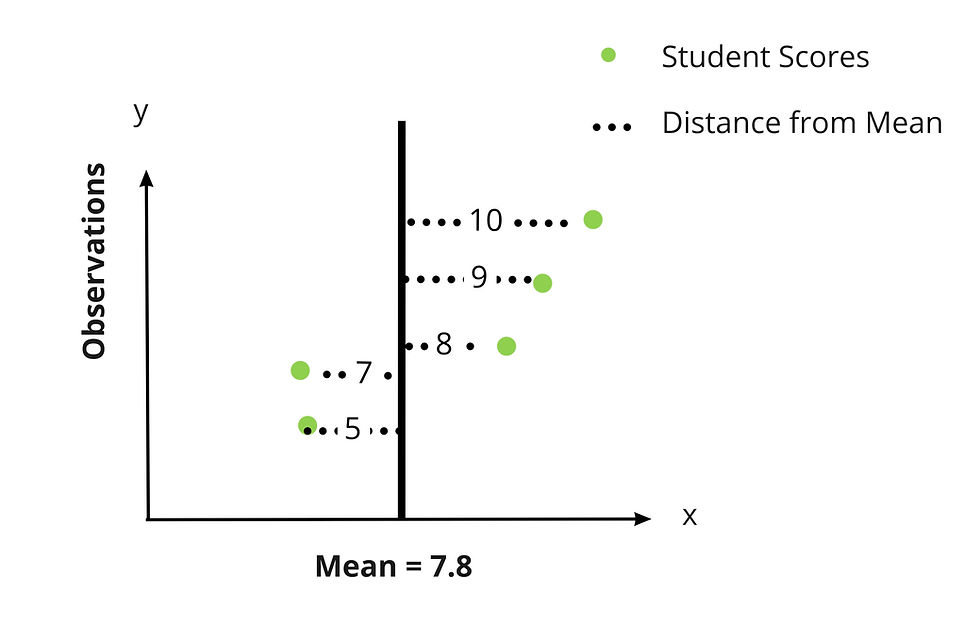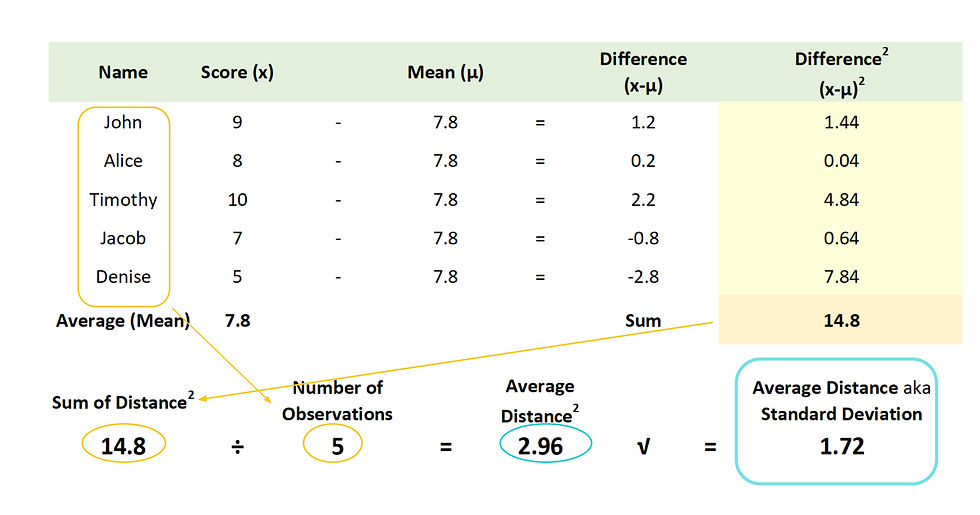top of page

# Mean & Standard DeviationMean and Standard Deviation are the two most important concepts to understand when learning statistics. Once you master them, everything else falls into place.

## Average

You're probably already familiar with what an average is. It is the most central or common value of a dataset.

There are three types of Average: Mean, Median, and Mode.

Mean - Most commonly used measure of average where you add up all the values in the dataset and divide by the number of data points (observations).

Median - Line up all of the data points from smallest to largest, and find the value (or average of the two values) in the center.

Mode - The value that occurs most frequently in a dataset. (Not all datasets have modes)

In statistics, a sample mean is denoted by the symbol, and a population mean is denoted by the μ symbol (samples are written using by Latin letters and populations are written using Greek letters).

## Standard Deviation

Standard deviation is the most important concept in statistics. It is the average distance between the observations in your dataset.

A sample standard deviation is denoted by the s symbol, and a population standard deviation is denoted by the σ symbol.

When you think standard deviation - think difference or change. It's a measure of the difference or change that is happening within a dataset.The formula for Standard Deviation is:(Don't let it scare you - we'll show you how to solve it!)

For example, here is how we found the standard deviation for a data set of student test scores:## Solving the Standard Deviation Equation:The standard deviation of a dataset is found by:

1. Finding the average of the data points (population mean)

2. Finding the difference between each data point and the mean

3. Squaring the differences

4. Summing the squared differences

5. Dividing by the number of number of observations (data points)

6. Calculating the square root

Notice that if you add up the differences before squaring them, they add up to zero! (The positive and negative values cancel out.) That is why we have to square them - so that they are all positive numbers. We reverse this by taking the square root in the end.